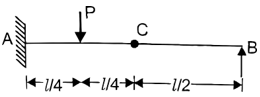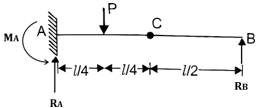Engineering Jobs   »   Civil Engineering Quiz

# GATE’22 CE : Weekly Practices Quiz: 18.07.2021

Quiz: Civil Engineering
Exam: GATE
Topic: Miscellaneous

Each question carries 2 marks
Negative marking: 1/3 mark
Time: 20 Minutes

Q1. For the propped cantilever shown in the figure, a hinge is provided at C. A and B are at the same level. What is the force reaction at the fixed end A?(a) (4/3) P
(b) P
(c) (3/4) P
(d) P/2

Q2. What is the nearest magnitude of strength of a 6 mm fillet weld of 100 mm length made between two flats each 10 mm thick? The allowable shear stress on the weld is 110 MPa
(a) 23 kN
(b) 33 kN
(c) 46 kN
(d) 66 kN

Q3. The moisture content of a certain municipal solid waste with the following composition will be

 Wet, % weight Dry, % weight Food waste 10 03 Paper 35 30 Yard waste 20 10 Others 35 20

(a) 100%
(b) 63%
(c) 37%
(d) 13%

Q4. A theodolite was set up at P and the angle of elevation to the top of a mobile tower ST was 30°. The staff reading held at a station of RL 110 m was 2.555 m, the telescope being horizontal distance between the foot of the tower and the instrument station is 810 m. the RL of the top of the tower is
(a) 578.25 m
(b) 579.50 m
(c) 580.25 m
(d) 582.40 m

Q5. A bar of 40 mm diameter and 400 mm length is subjected to an axial load of 100 kN. It elongates by 0.150 mm and that the diameter decreases by 0.005 mm. what is the Poission’s ratio of the material of the bar?
(a) 0.37
(b) 0.35
(c) 0.33
(d) 0.30

Q6. A bolt designated as Hex bolt M16 × 70NL will have
(a) Cross – section area of 16 × 70 〖cm〗^2
(b) Length of 16 mm
(c) Diameter of 70 mm
(d) Diameter of 16 mm

Q7. A test plate 30 cm × 30 cm resting on a sand deposit settles by 10 mm. under a certain loading intensity. A footing 150 × 200cm. resting on the on the same sand deposit and loaded to the same load intensity settles by
(a) 50 mm
(b) 30.2 mm
(c) 27.8 mm
(d) 2.0 mm

Q8. A lighthouse is visible just above the horizon at a certain station at the sea level. The distance between the station and the lighthouse is 40 km. the height of the lighthouse is approximately
(a) 187 m
(b) 137.7 m
(c) 107.7 m
(d) 87.3 m

Q9. Match List-I with List-II and select the correct answer:

 List-I List-II A.      Serviceability B.      Shear key C.      Shrinkage D.     Concrete spalling 1.      Sliding 2.      Deflection 3.      Cracking 4.      Corrosion

Codes:

A          B          C          D

(a)        1          3          4          2

(b)        2          1          3          4

(c)        1          3          2          4

(d)        2          1          4          3

Q10. The average daily traffic on a stretch of road is 300 commercial vehicles per lane per day Design traffic repetition for 10 years when vehicle damage factor is 2.5 and traffic growth rate is 7%, is
(a) 3.8 msa
(b) 23.5 msa
(c) 45.4 msa
(d) 16 msa

Solutions
S1. Ans.(b)
Sol.ΣV=0
R_A+R_B=P——-(1)
ΣM_C=0
R_B×L/2=0
▭(R_B=0)
From equation (1)
▭(R_A=P)

S2. Ans.(c)
Sol. Fillet size (f_S )=6mm.
Length (L) = 100 mm.
Allowable shear stress (P_ss )=110 MPa
Strength of weld (P) = 0.7 × f_s×L×P_ss
=0.7×6×100×110
=46,200 N
▭(P=46.2 kN)

S3. Ans.(c)
Sol. Total weight = 10 + 35 + 20 + 35 = 100 units
Dry weight = 03 + 30 + 10 + 20 = 63 units
Available moisture = 100 – 63 = 37 units
%Moisture content = 37/100×100% = 37%

S4. Ans.(c)
Sol.From ∆APQ
Tan 30°=h/810
h=810×1/√3
▭(h=467.65m)
RL of top of tower = RL of station + BS + h
= 110 + 2.555 + 467.65
= 580.208 m

S5. Ans.(c)
Sol. Given,
d = 40mm
∆d= -0.005 mm.
L=400 mm
∆L=0.150mm
μ= ?
Poission’s ratio (μ)=-(Latrel strain)/(Longitudinal strain)
=-((∆d/d))/((∆L/L) )
= -(((-0.005)/40))/((0.150/400) )
=1/3
▭(μ=0.33)

S6. Ans.(d)
Sol. Hex bolt designation M16 ×70 NL means bolt have nominal diameter of 16 mm and length of 70 mm.

S7. Ans.(c)
Sol.
S_P=10 mm.
B_P=0.3 m
B_F=1.5 m
S_F= ?
For sandy soils
S_P/S_F =[(B_P (B_F+0.3))/(B_F (B_P+0.3) )]^2
10/S_F =[0.3(1.5+0.3)/1.5(0.3+0.3) ]^2
▭(S_F=27.8 mm)

S8. Ans.(c)
Sol. Given, d = 40 km
Height of lighthouse (h) will be –
h=0.0673 d^2
=0.0673×(40)^2
=107.68 m.

S9. Ans.(b)
Sol. Serviceability → Deflection
Shear key → Sliding
Shrinkage → Cracking
Concrete spalling → corrosion
→ Concrete spalling is caused by corrosion of steel reinforcement bars.

S10. Ans.(a)
Sol. Given, n=10
A=300
r=7%=0.07
F=2.5
N_s=?
N_s=365A[((1+r)^n-1)/r]×F
=365×300[((1+0.07)^10-1)/0.07]×2.5
▭(N_s=3.8 msa)

Sharing is caring!

Thank You, Your details have been submitted we will get back to you.